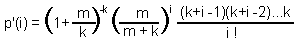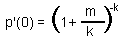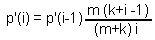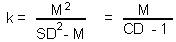## 3.3. Aggregated spatial distributions

There is no universal theoretical model for aggregated spatial distributions. However, there are empirical models, e.g., negative binomial distribution (NBD) which work very well in the majority of cases. NBD is described by the equation:where m is mean, k is "coefficient of aggregation" (aggregation increases with decreasing k). The zero term (the proportion of empty samples) equals to:Other terms can be estimated by iteration:Estimating NBD parameters using the method of moments:

m = MFor a random distribution, CD=1 and k is infinite. When k increases to infinity then NBD distribution coincides with poisson distribution.

Alexei Sharov 12/28/95